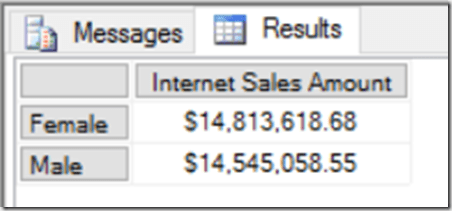# Excel Cube Functions, Dynamic Arrays And Lambdas, Part 3: Grouping And Histograms

In the last post in this series I showed how you can use Excel’s new Lambda helper functions to return tables. In this post I’ll show you how you can use them to return a dynamic array of CubeSet functions which can be used to build a histogram and do the kind of ABC-type analysis that can be difficult to do in a regular Power BI report.

For the examples in this post I added some rows to the Excel Data Model table that I’m using to hold my source data:

The aim here is to put these products into an arbitrary number of groups, or buckets, based on their sales. To define these buckets I created another Excel table called Buckets that has three columns: the name of the bucket, and the lower bound and the upper bound of the sales amount that determines whether a product should fall into the bucket:

I then created two dyanmic array formulas using the new Map function. In cell G2 I added this formula:

```=
MAP(
Buckets[Bucket Name],
Buckets[Lower Bound],
Buckets[Upper Bound],
LAMBDA(
n,
l,
u,
CUBESET(
"ThisWorkbookDataModel",
"FILTER([Sales].[Product].[Product].MEMBERS, [Measures].[Sales Amount]>=" & l &
" AND [Measures].[Sales Amount]<=" & u & ")",
n)
)
)
```

And in cell H2 I added this formula:

```MAP(
G2#,
LAMBDA(
s,
IF(
CUBESETCOUNT(s)>0,
CUBEVALUE(
"ThisWorkbookDataModel",
s,
"[Measures].[Sales Amount]"),
0)
)
)

```

Here’s what these two formulas return:The formula in G2 takes three arrays – the values from the three columns in the Buckets table – and then loops over the values in those columns and uses the CubeSet function to return a set of the Products whose sales are between the lower and upper bounds. Since there are two rows in the Buckets table, this formula returns two sets. The formula in H2 uses the CubeValue function to return the aggregated sales amount for each set.

Last of all I created a column chart bound to the values in G2 and H2. This was a bit tricky to do, but I found the answer in this video from Leila Gharani – you need to create names that return the contents of the ranges G2# and H2# and then use the names in the chart definitions.

The beauty of all this is what when you edit the ranges in the Buckets table in the top left of the worksheet, edit the names of the buckets or add new buckets, the table and chart update automatically.

After doing all this I realised there was another, probably easier way to achieve the same result without using the Map function. All I needed to do was to add new calculated columns to the bucket table to return the sets and values:

Here’s the formula for the Set column in the table above:

```=CUBESET(
"ThisWorkbookDataModel",
"FILTER([Sales].[Product].[Product].MEMBERS, [Measures].[Sales Amount]>=" &
[@[Lower Bound]] &
"AND  [Measures].[Sales Amount]<=" &
[@[Upper Bound]] &
")",
[@[Bucket Name]] &
" set"
)

```

…and here’s the formula for the Sales column in that table:

```= IF(
CUBESETCOUNT(
[@Set])>0,
CUBEVALUE(
"ThisWorkbookDataModel",
[@Set],
"[Measures].[Sales Amount]"
),
0
)

```

I think this second approach should work with any version of Excel since the introduction of tables and cube formulas.

# Excel Cube Functions, Dynamic Arrays And Lambdas, Part 2: Returning Tables

In the first post in this series I showed how to use the new Excel Lambda helper functions to return an array containing all the items in a set. That isn’t very useful on its own, so in this post I’ll show you how to generate an entire dynamic table using Excel cube functions and Lambda helper functions.

In this post I’ll be using the same source data as in my previous post: a table containing sales data with just two columns.

With this table added to the Excel Data Model/Power Pivot, I created two measures:

I then created created two sets using CubeSet containing the sets of Products (in cell B2 of my worksheet) and Measures (in cell B4) to use in my table:

```=CUBESET("ThisWorkbookDataModel", "[Sales].[Product].[Product].MEMBERS", "Product Set")

=CUBESET("ThisWorkbookDataModel", "{[Measures].[Sales Amount], [Measures].[Forecast Sales]}", "Measure Set")
```

Here are the formulas shown in the worksheet:

And here’s the output – remember you only see the text in the third parameter displayed in the cell:

Now, here’s the fun part – a single formula that takes these sets and builds a table with the Measures on columns and the Products on rows:

```=MAKEARRAY(
CUBESETCOUNT(B2)+1,
CUBESETCOUNT(B4)+1,
LAMBDA(r,c,
SWITCH(
TRUE(),
AND(r=1,c=1),
"",
c=1,
CUBERANKEDMEMBER("ThisWorkbookDataModel",\$B\$2,r-1),
r=1,
CUBERANKEDMEMBER("ThisWorkbookDataModel",\$B\$4,c-1),
CUBEVALUE("ThisWorkbookDataModel",
CUBERANKEDMEMBER("ThisWorkbookDataModel",\$B\$2,r1),
CUBERANKEDMEMBER("ThisWorkbookDataModel",\$B\$4,c-1)
)
)
)
)
```

Here’s what this formula returns:

How does this work? Going through the MakeArray function step-by-step:

• The first two parameters specify that the output will be an array with one more row than there are items in the Product set and one more column than there are items in the Measures set.
• The third parameter returns a Lambda that is called for every cell in this array. This Lambda contains a Switch with the following conditions:
• For the top-left cell in the array, return a blank value
• In the first column, use the CubeRankedMember function to return the Products on the rows of the table
• In the first row, use the CubeRankedMember function to return the Measures on the columns of the table
• In the body of the table, use the CubeValue function to return the values

Here’s a slightly more ambitious version that returns the same table but adds a total row to the bottom:

```=
LET(
NumberOfRows,
CUBESETCOUNT(B2)+2,
NumberOfColumns,
CUBESETCOUNT(B4)+1,
MAKEARRAY(
NumberOfRows,
NumberOfColumns,
LAMBDA(r,c,
SWITCH(
TRUE(),
AND(r=1,c=1),
"",
AND(r=NumberOfRows,c=1),
"Total",
r=NumberOfRows,
CUBEVALUE("ThisWorkbookDataModel",
\$B\$2,
CUBERANKEDMEMBER("ThisWorkbookDataModel",\$B\$4,c-1)),
c=1,
CUBERANKEDMEMBER("ThisWorkbookDataModel",\$B\$2,r-1),
r=1,
CUBERANKEDMEMBER("ThisWorkbookDataModel",\$B\$4,c-1),
CUBEVALUE("ThisWorkbookDataModel",
CUBERANKEDMEMBER("ThisWorkbookDataModel",\$B\$2,r-1),
CUBERANKEDMEMBER("ThisWorkbookDataModel",\$B\$4,c-1))
)
)
)
)
```

Two extra things to note here:

• This is a great example of a complex formula where the new Excel Let function can be used to improve readability and prevent the same value being evaluated twice.
• The values in the Total row are calculated in the Excel Data Model, not on the worksheet, by using the CubeSet function inside the CubeValue function. This means that the totals will be consistent with what you see in a PivotTable and therefore correct

This is still very much a proof-of-concept. I need to look at the performance of this approach (it may not be optimal and may need tuning), and I’m not sure how a table like this could be formatted dynamically (especially the Total row). It is exciting though!

# Excel Dynamic Arrays And The CubeSet Function

Among all the exciting announcements made at Ignite last week, one you may have missed (even in all the Excel-related announcements here) was that dynamic arrays have finally reached GA. Ever since they were announced I’ve been interested in how they can be used with Excel cube functions, which allow you to get data from Analysis Services, Power BI and the Excel Data Model/Power Pivot into cells on the worksheet, and I’ve blogged about this once already. Even though right now the CubeValue function can’t be used with dynamic arrays – alas – there are still some interesting ways dynamic arrays and cube functions can be used together and in this post I’ll show you another one I’ve found.

Say you have the following set of tables in the Excel Data Model:And the following measure that counts the number of customers who bought something:

```Distinct Customers:=DISTINCTCOUNT('Internet Sales'[CustomerKey])

```

Let’s also say you have on a worksheet the following table of data showing the number of distinct customers broken down by product, created using the CubeMember and CubeValue functions: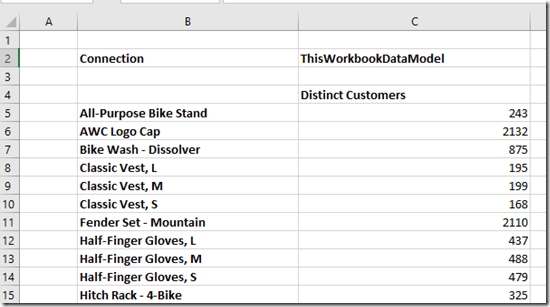Here are the formulas for this table:So far, all very straightforward. Now let’s say you want to use a dynamic array to filter the rows in this table so you only see the products that have more than a certain number of distinct customers, and you want to see a grand total for this filtered list of customers, like so: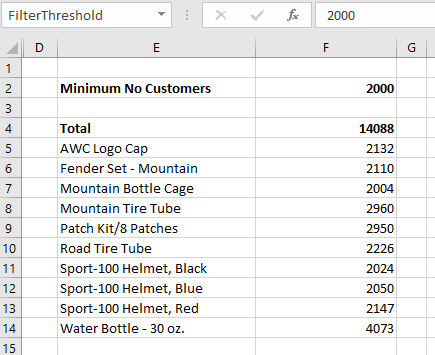Here are the formulas to achieve this:To explain what’s going on here:

• Cell C2 contains the text “ThisWorkbookDataModel”, the name of the connection to the Excel Data Model. Doing this makes the other Excel formulas here much easier to read!
• Cell F2 – which I’ve turned into a named range called FilterThreshold – contains the number of customers which a product must exceed to be shown.
• Cell E5 contains the dynamic array Filter function that filters the rows shown in the first two screenshots above and only returns the products that exceed the threshold. The formula is:
FILTER(B5:B134,C5:C134>FilterThreshold)
• Cell E6 does something similar to E5 but returns the number of distinct customers for each product. If all I wanted was the products and the number of distinct customers I wouldn’t have needed two separate formulas, I could have used one, but I need to add the grand total too…
• Cell E4 contains the formula:
CUBESET(\$C\$2, E5#, “Total”)
This creates a set from the spill reference E5#, which contains the cells containing CubeMember formulas returned by the Filter function. For some reason CubeSet returns an error if you try to use a dynamic array formula in its second parameter but using a spill reference works ok; this is why I had to use separate formulas in E5 and E6.
• Finally, cell F4 contains the formula that returns the total distinct count for all products returned in the filtered list using CubeValue and the set created in cell E4:
CUBEVALUE(\$C\$2, “[Measures].[Distinct Customers]”, E4)
Since this is a distinct count the only way to get the correct value here is using CubeSet, CubeValue and the DAX measure – there would be no way to calculate the correct value using Excel formulas. The same could be said of almost any other DAX measure that wasn’t a simple aggregation.

# Obscure MDX Month: Deselecting Members In An Excel PivotTable Leads To Missing Rows

Here’s some interesting (and borderline buggy) Excel PivotTable behaviour I learned about today from Charles-Henri Sauget, as well as the workaround for it courtesy of the great Greg Galloway.

Say you have a large dimension attribute hierarchy with 200,000 members on it in SSAS MD (or the equivalent in Tabular or Power Pivot) and drop it onto the rows of an Excel PivotTable. As you would expect, you get a PivotTable with 200,000 rows in it:However if you then deselect just one member on rows like so:…you’ll find that the PivotTable does not have 199,999 rows – in Excel 2016 it only has 32,000 rows:(different versions of Excel may return different numbers of rows here, but still not the full number).

If you look at the MDX generated by Excel it consists of all of the member unique names that are still selected, and unsurprisingly it’s a gigantic query:However, it turns out you can make Excel do the sensible thing and use the Except() function to return everything apart from the deselected member by going to the Field Settings dialog and selecting “Include new items in manual filter”: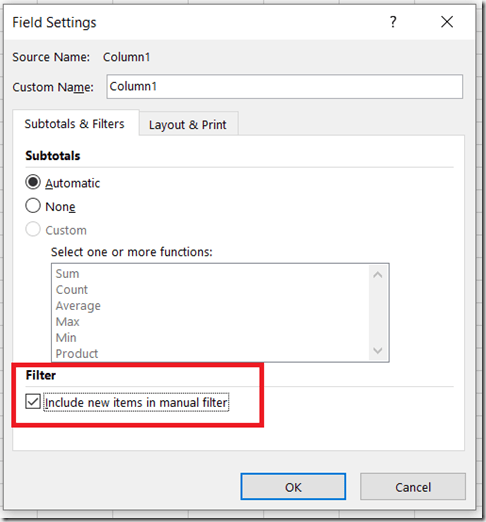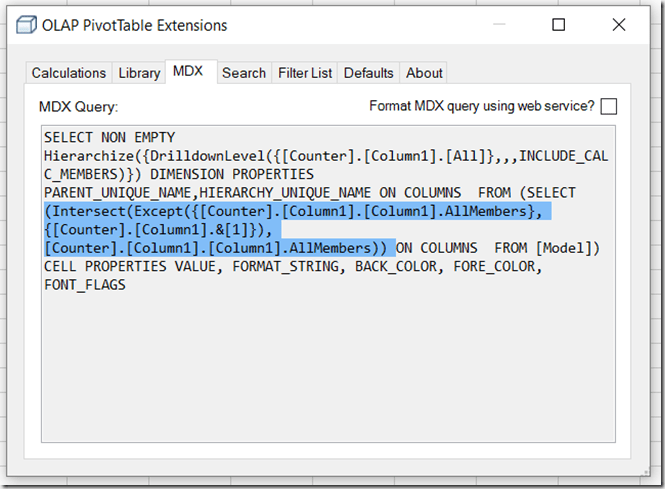This then gives you the expected number of rows in the PivotTable:I suspect the reason Excel is generating the crazy-long MDX statement by default is that it’s the only way to prevent new members being added to the PivotTable if they are added to the attribute hierarchy in future. On a really large attribute hierarchy, though, the risk is that the resulting MDX query might exceed the maximum length of a query, so Excel has to truncate the number of members returned to make the query shorter. With “Include new items in manual filter” selected, though, it’s ok if new members do get added to the PivotTable in the future so it’s ok to use the Except() function in the query.

# Handling Missing Members In The CubeSet() Function With Power Pivot

Last week I received an email from a reader asking how to handle missing members in MDX used in the Excel CubeSet() function. My first thought was that this could be solved easily with the MDXMissingMemberMode connection string property but it turns out this can’t be used with Power Pivot in Excel 2013/6 because you can’t edit the connection string back to the Excel Data Model: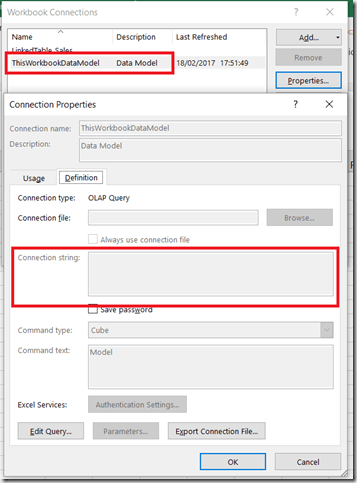Instead, you have no choice but to handle this in MDX.

Here’s an illustration of the problem. Imagine you have the following table of data on your Excel worksheet:With this table added to the Excel Data Model, you could write the following Excel formula using CubeSet():

```=CUBESET(
"ThisWorkbookDataModel",
"{[Sales].[Product].[All].[Apples],
[Sales].[Product].[All].[Oranges],
[Sales].[Product].[All].[Pears]}",
"Set")
```In the screenshot above the CubeSet() formula is used in H3, while in H4 there’s a formula using CubeSetCount() that shows the set contains three members.

If the source data is updated so that the row for Pears is deleted like so:Then the CubeSet() formula returns an error because the member Pears no longer exists:How can this be avoided? If what you actually wanted was all of the Products, whatever they were, the best thing to do is to use the MDX Members function like so:

```=CUBESET(
"ThisWorkbookDataModel",
"{[Sales].[Product].[Product].MEMBERS}",
"Set")
```

[I talk about the Members function in this post in my series of posts on MDX for Power Pivot users]

This formula does not return an error and you can see that the CubeSetCount() formula below shows the set only contains two members now:If you do need to refer to individual members then the MDX you need is more complicated:

```=CUBESET(
"ThisWorkbookDataModel",
"{[Sales].[Product].[All].[Apples],
[Sales].[Product].[All].[Oranges],
iif(
iserror(
strtomember(""[Sales].[Product].[All].[Pears]"")
),
{},
{strtomember(""[Sales].[Product].[All].[Pears]"")}
)
}",
"Set")
```This MDX uses the StrToMember() function to interpret the contents of a string as an MDX expression returning a member; if this expression returns an error then it is trapped by the IsError() function and an empty set is returned.

This isn’t particularly pretty, though, and ideally the MDXMissingMemberMode connection string property would be set to Ignore in the Excel Data Model connection string property.

# Power BI Model Size Bloat And Auto Date/Time Tables

Opinion is split over Power BI’s ability to automatically create Date hierarchies in your model. Personally it drives me mad and I always turn it off, but I know a lot of people love the convenience of it. Whatever your feelings, though, it is important to be aware of the problems it can cause with the size of your model.

Imagine you have a .pbix file and you load just this one table into the data model:Three columns, each containing three dates with long gaps in between, but only nine values overall. No other tables or queries, no measures, no visuals at all. When you save it results in a file that is a massive 4.7MB – but why, when there’s hardly any data?

Actually, there is a lot of data hidden in this file. If you connect to the .pbix file with DAX Studio you can see that the Auto Date/Time functionality has built three hidden Date tables whose names are prefixed with “LocalDateTable”, one for each date column in the original table above:These tables can be queried in DAX Studio, and the following query reveals more about them (if you try this on your model you will need to alter the name of the table used in the query to match the names of one of the tables in your model):

```EVALUATE
ROW (
"Hidden Date Table Rowcount",
"Min Date",
"Max Date",
)
```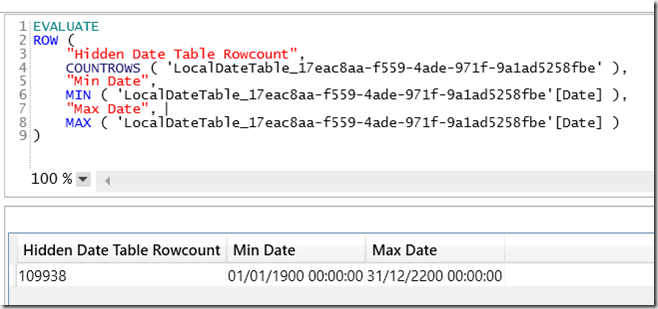In this case each of the three tables has 109938 rows. That’s one row for each date between the beginning of the year containing the earliest date in the source column and the end of the year containing the latest date in the source column – which is the best practice for building a Date table, but results in three very large tables in this case.

To stop Power BI automatically building these tables for you, in Power BI Desktop go to the File menu, select Options, then Data Load and deselect the Auto Date/Time option: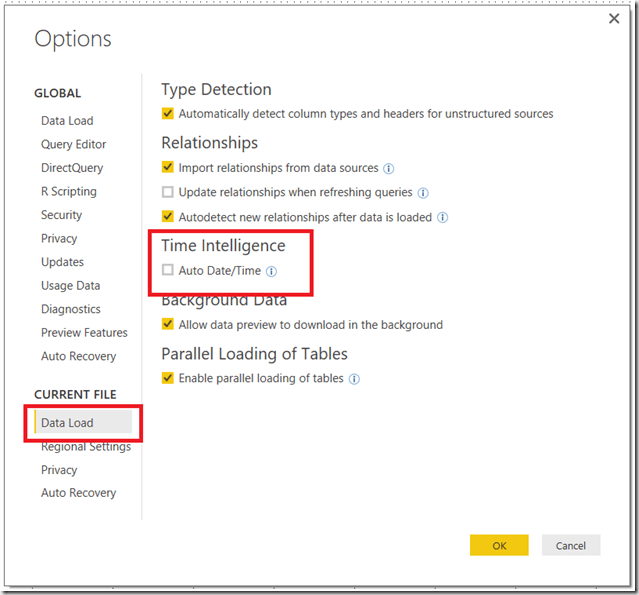When you do this the automatically created date tables are removed from the model. In this case, after saving, the .pbix file shrinks to 181KB! Remember that, by doing this, you won’t get automatic date hierarchies created for you when you are designing your reports and you will have to build any Date tables and hierarchies you need manually.

This is an extreme example of course, but overall you should probably turn off Auto Date/Time if your model size is an issue and:

• You have a lot of date columns in your tables, or
• Your date columns contain large ranges of dates. Remember that some systems use 1/1/1900 as an ‘unknown’ date value, which can make things a lot worse.

# Nested Variables In DAX

Last week, at the SQL Server Days conference in Belgium, Kasper mentioned in his presentation that it was possible to define variables inside variables in DAX. So, for example, you could define a measure like so:

```MyMeasure =
var Outer1 =
var Inner1 = 1
var Inner2 = 2
return Inner1 + Inner2
var Outer2 = 3
return Outer1 + Outer2

```

This measure returns 6 as you might expect: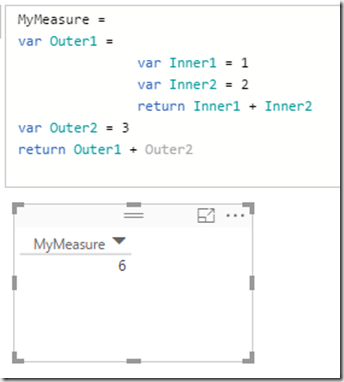There aren’t any performance benefits to doing this, although of course it helps with code readability and organisation (thanks to Marius for confirming this).

With my newly rekindled love of DAX I thought this was quite interesting. I’m not really sure why though, given that it’s not particularly useful; I think Matt might be right:# Using The CubeSet() Function to Combine Selections From Multiple Slicers in Excel Power Pivot Reports

Since my last post on the Excel cube functions proved to be very popular, I thought I’d write a follow-up that shows off some more little-known but interesting functionality: how to use the CubeSet() function to combine selections from multiple slicers.

The Problem

Consider the following Power Pivot model based on data from the Adventure Works DW database:The Internet Sales table contains one row per sales transaction; Product and Date are dimension tables that provide more information on the product bought in the transaction and the date the order was taken on. There’s one measure called Distinct Customers that returns the distinct count of customers; its definition is as follows:

```Distinct Customers:=DISTINCTCOUNT('Internet Sales'[CustomerKey])

```

With this model you could build the following simple report by converting a PivotTable to cube functions:Obviously this will tell you how many distinct customers bought the products selected in the slicer each year. You could also duplicate the slicer and the cube functions and have two reports side-by-side so you could compare the number of customers that bought two different groups of products:The question here is, though, how can you create a third report that shows the number of distinct customers that bought any of the products selected across both slicers? So, using the example above, the report on the left shows the number of customers that bought Mountain-200 Black 38, Mountain-200 Black 42 or Mountain-200 Black 46; the report on the right shows the number of customers that bought Mountain-200 Silver 38, Mountain-200 Silver 42 or Mountain-200 Silver 46; the aim is to create a third report that shows the number of distinct customers that bought Mountain-200 Black 38, Mountain-200 Black 42, Mountain-200 Black 46, Mountain-200 Silver 38, Mountain-200 Silver 42 or Mountain-200 Silver 46 without having to select all those products in a third slicer.

The Solution

Step 1

The first thing to point out is that you can use the CubeSet() function to return the set of items selected in an Excel slicer. This is a widely-known technique – Rob Collie has a great post describing it here. What I didn’t realise until recently though was that one CubeSet() function can point to other cells containing CubeSet() functions and union all the items returned by those CubeSet() functions. This allows you to combine the selections made in multiple slicers, assuming that those slicers refer to the same field in your Power Pivot model. Here are the formulas in three cells that do this: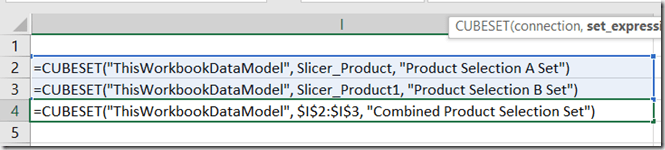In cell I2 the formula uses the CubeSet() function to capture the selection made in the left-hand slicer in the report above, captioned Product Selection A, which has the name Slicer_Product. In cell I3 there is a similar formula to capture the selection made in the slicer on the right in the report, captioned Product Selection B, which has the name Slicer_Product1. The formula in I4 then uses a third CubeSet() function to combine the sets returned by the last two formulas into a third set.

Step 2

Now that you have a single CubeSet() formula in I4 that combines the selections in both slicers, you can use the trick I showed in my last post and reference that cell in CubeValue() formulas to get the number of distinct customers that bought any of the products listed in either slicer: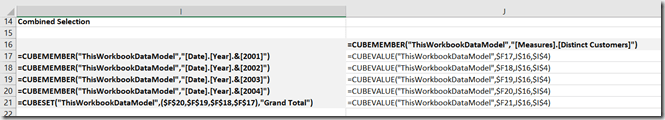Here’s what the report looks like at this point: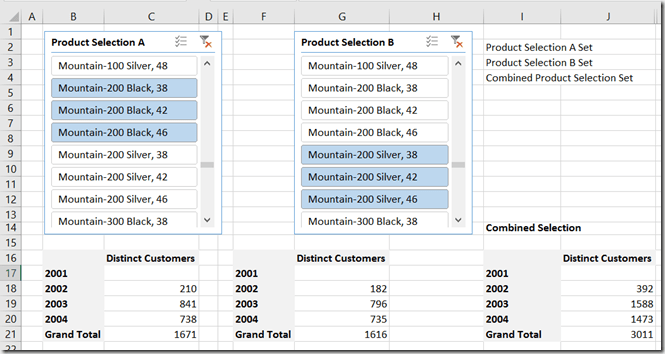Step 3

Notice that, thanks to the power of Power Pivot, customers are not double-counted in the new combined selection report on the right-hand side. For example, in 2003 841 customers bought one or more products selected in the slicer Product Selection A while in 2003 796 customers bought one or more products listed in the slicer Product Selection B. 841+796=1637, but in the combined selection report correctly states that only 1588 distinct customers bought products listed in either Selection A or Selection B. We can use these numbers to do one final, useful calculation: 1637-1588=49, so there were 49 customers who must have bought products in both Selection A and Selection B. The formula to do this is pretty straightforward and looks like this:

```=IFERROR((\$C19+\$G19)-\$J19, 0)

```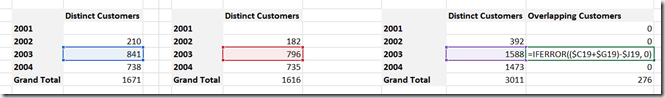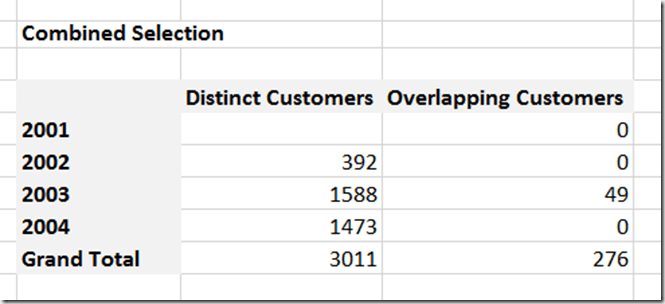# Creating OR Filters With Cube Functions For Power Pivot And Analysis Services Reports In Excel

The Excel Cube Functions are incredibly powerful, and I’m still amazed at the kind of problems they can solve. This post describes how they can be used to build a report with a complex OR filter using data from Power Pivot (it’s equally applicable to SSAS) that shows a number of advanced uses of these functions. No knowledge of MDX or DAX is needed but if you’re new to the Excel Cube Functions I recommend that you watch this video of a presentation by Peter Myers, which provides an excellent introduction to them.

The Problem

Imagine you’ve got a very simple Power Pivot model that looks like this:There’s a Date table with dates, months and years in, and an Internet Sales table with sales data in and a measure called Sales Amount. Here’s what the data looks like in a PivotTable: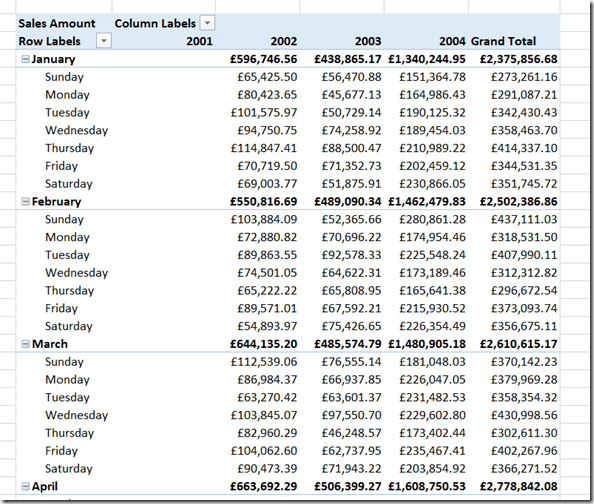Now, imagine that you want a report with the Sales Amount measure on columns and Years on rows, and you want to filter the data so that you only see values for Mondays in July or Wednesdays in September. Using the Fields, Items and Sets functionality you could filter the data to only show the day/month combinations you need for each year, but since you can’t put a named set into the Filter area of a PivotTable you would have to use Excel formulas to sum up the combinations to get the totals you need:Lukcily it is possible to build the report you need using the Cube Functions! Here’s how:

Step 1: Build Your Combinations Using CubeMember()

The first thing to point out is that the CubeMember() function does not have to just return a member, it can return a combination of members (in MDX this is known as a tuple). These combinations can be built in several ways, one of which is by using cell references to other cells that themselves contain CubeMember() functions. It’s probably easier to explain this by showing a worksheet that contains six cells with CubeMember() functions in. Here it is with the formulas visible:Cells B5 and B10 contain references to days of the week; cells B6 and B11 contain references to months. Cells B7 and B12 contain CubeMember() functions that return the combinations we want to filter by: Mondays in July and Wednesdays in September respectively.

Here’s what the formulas above return:You’ll notice that the ‘combination’ cells only show the month names, not the day/month combinations – this is just a feature of the CubeMember() function and can be a bit misleading, but rest assured they do return the combinations you need.

Step 2: Build A Set Using CubeSet()

The CubeSet() function is also able to build sets using cell references to cells containin CubeMember() functions. In this case I want a set containing the two ‘combination’ CubeMember() functions from B7 and B12. I can do this by using the formula:

```=CUBESET(\$B\$2,(\$B\$7,\$B\$12), "The set of combinations")
```

Here are the formulas on the worksheet at this point: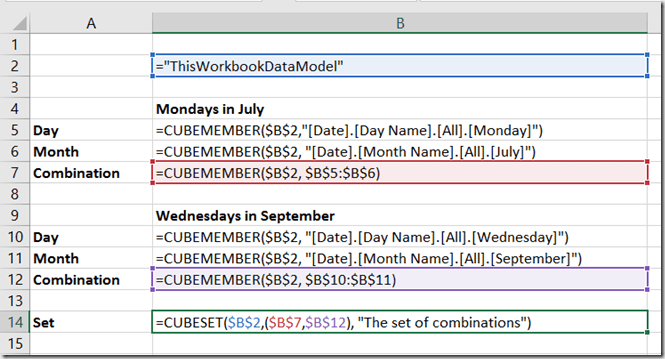And here’s the output:Step 3: Reference The CubeSet() Function In Your Report

Now you have a CubeSet() function that returns the two day/month combinations, you can use this in a cube function report. When you reference a cell containing the CubeSet() function in a CubeValue() formula, the CubeValue() formula will return the aggregated value of all of the combinations in the CubeSet(). So for example, here’s a report with the Sales Amount measure on columns, Years on rows, and displaying the Sales Amount for each year filtered by the two day/month combinations:And here’s the actual output: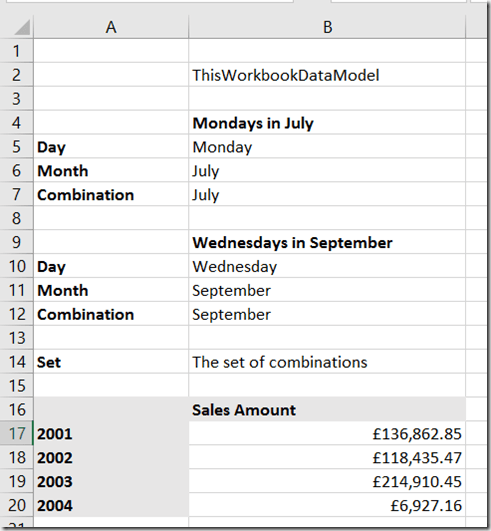Compare the numbers from the report at the bottom with the values calculated from the PivotTable in the screenshot earlier in this post, and you’ll see that we have indeed shown just the combined Sales Amount for Mondays in July and Wednesdays in September, broken down by Year.

You can download the example Power Pivot workbook for this post here.

# Excel 2016 PivotTable MDX Changes Lead To Big Query Performance Gains

Yesterday, the Excel team announced a bunch of performance improvements in Excel 2016 for PivotTables connected to Analysis Services (Multidimensional or Tabular), Power Pivot and Power BI. If you haven’t read the official blog post already, here it is:

https://blogs.office.com/2016/07/07/faster-olap-pivottables-in-excel-2016/

In that post, Microsoft point out that how much of a performance increase you get will depend on a number of factors. I guess they have to do this to manage expectations. However I’m going to come right out and say that these changes are probably the most important thing that has happened in the last five years for Analysis Services or Power Pivot query performance and if you are using Excel PivotTables with SSAS or Power Pivot for reporting, you should upgrade to Excel 2016 (you’ll need the Office 365 click-to-run version) just for these changes.

The key improvement that Microsoft have made here is to stop Excel bringing back unnecessary subtotal values in the MDX queries that it generates. This has been a gigantic problem for years and several people have blogged about it: I did here, and among other people Rui Quintino and Richard Lees have posts on the subject that are worth reading. A lot of my consultancy work involves SSAS and MDX performance tuning and I think I see around five to ten customers every year who are seriously affected by this – and I’m sure this is just the tip of the iceberg. Even if you don’t think you are affected, you may have users who are putting up with slow refresh times for Excel-based reports without your knowledge.

Let’s go into some technical detail, see what the original problem was and what has changed in Excel 2016. I’m going to provide some examples using Excel 2013/2016 against SSAS Multidimensional but as I said the same problems occur (although less severely) with SSAS Tabular and Power Pivot.

Consider the following Excel 2013 PivotTable connected to the Adventure Works cube:It’s typical of the kind of thing an end user wants to build, and there are two things to point out:

1. Notice there are three fields that have been placed on the Rows axis of the PivotTable: the Gender and Marital Status hierarchies from the Customer dimension, and the Category hierarchy from the Product dimension. It’s pretty common for users to put many more fields together on an axis when they want to display detailed data.
2. The user has also turned off grand totals and subtotals in the PivotTable so that only the detailed data is shown. This often happens in combination with (1) because if the user only wants to see the detailed data the subtotals and grand totals are a distraction.

In this case the PivotTable layout has been switched Tabular and the Repeat All Item Labels option is selected to make the PivotTable look like a table, but these are just aesthetic changes.

This PivotTable contains 12 rows, but if you look at the MDX query that is generated by Excel 2013 to populate it, it returns 36 rows of data (actually the MDX in this case returns 36 columns, but that’s a quirk of Excel) because the subtotals and grand total that Excel is not displaying are still being returned. Here’s the query:

```SELECT
NON EMPTY
CrossJoin(
CrossJoin(
Hierarchize(
{DrilldownLevel(
{[Customer].[Gender].[All Customers]}
,,,INCLUDE_CALC_MEMBERS)}),
Hierarchize(
{DrilldownLevel({[Customer].[Marital Status].[All Customers]}
,,,INCLUDE_CALC_MEMBERS)})),
Hierarchize(
,,,INCLUDE_CALC_MEMBERS)}))
DIMENSION PROPERTIES PARENT_UNIQUE_NAME,HIERARCHY_UNIQUE_NAME
ON COLUMNS
FROM
WHERE ([Measures].[Internet Sales Amount])
CELL PROPERTIES VALUE, FORMAT_STRING,
LANGUAGE, BACK_COLOR, FORE_COLOR, FONT_FLAGS

```

And here’s a sample of what it returns, with the unwanted values highlighted:You can probably guess that these unwanted subtotals and grand totals make the query slower, and the more fields you put together on the rows or columns of a PivotTable the greater the number of subtotals/grand totals are returned and slower things get. PivotTables connected to SSAS or Power Pivot often become completely unusable with more than six or seven fields put together on the same axis, and the only workarounds before Excel 2016 are either to write custom MDX in a named set (I show how to do this here) or if you are using SSAS Multidimensional try to use member properties instead of separate fields (as Richard Lees shows here), but neither are really great alternatives.

Building the same PivotTable using Excel 2016, however, gives the following MDX:

```SELECT
NON EMPTY
CrossJoin(
CrossJoin(
Hierarchize(
{[Customer].[Gender].[Gender].AllMembers}),
Hierarchize(
{[Customer].[Marital Status].[Marital Status].AllMembers})),
Hierarchize(
{[Product].[Category].[Category].AllMembers}))
DIMENSION PROPERTIES PARENT_UNIQUE_NAME,HIERARCHY_UNIQUE_NAME
WHERE ([Measures].[Internet Sales Amount])
CELL PROPERTIES VALUE, FORMAT_STRING,
LANGUAGE, BACK_COLOR, FORE_COLOR, FONT_FLAGS

```

Which returns just the data needed for the PivotTable:What has changed? Focusing on just the Gender hierarchy, the difference between the two queries is that in Excel 2013 the selection on Gender is given using the DrillDownLevel() function on the All Member of the hierarchy. Isolated and put in its own query, the set expression used looks like this:

```SELECT
{[Measures].[Internet Sales Amount]}
ON COLUMNS,
DrilldownLevel(
{[Customer].[Gender].[All Customers]}
,,,INCLUDE_CALC_MEMBERS)
ON ROWS
FROM

```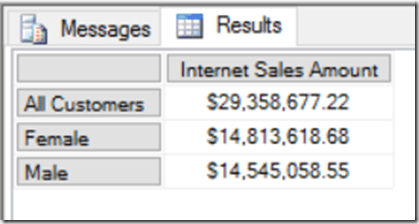Notice how the All Member, used by Excel to return subtotals and grand totals, is returned by the set. Excel 2016 instead just asks for the members on the Gender level of the Gender hierarchy, not including the All Member. Again, put inside its own query, you can see what it returns:

```SELECT
{[Measures].[Internet Sales Amount]}
ON COLUMNS,
{[Customer].[Gender].[Gender].AllMembers}
ON ROWS
FROM